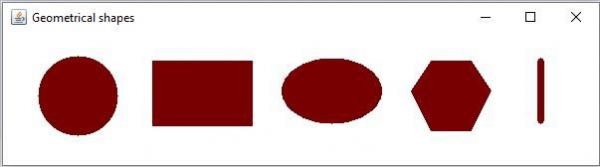# How to draw geometrical shapes on image using OpenCV Java Library?

The org.opencv.imgproc package of Java OpenCV library contains a class named Imgproc this class provies various methods such as, resize(), wrapAffine(), filter2D, to process an input image.

In addition to them It provides a set of method to draw geometrical shapes on images, Following are some of them −

ShapeMethod and Description
EllipseYou can draw an ellipse on an image using the ellipse() method.
CircleYou can draw a circle on an image using the circle() method.
RectangleYou can draw a rectangle on an image using the rectangle() method.
PolygonYou can draw a polygon on an image using the polygon() method.
LineYou can draw a line on an image using the line() method

## Example

Following Java example draws various shapes on a blank OpenCV image −

import org.opencv.core.Core;
import org.opencv.core.Mat;
import org.opencv.core.MatOfPoint;
import org.opencv.core.Point;
import org.opencv.core.RotatedRect;
import org.opencv.core.Scalar;
import org.opencv.core.Size;
import org.opencv.imgcodecs.Imgcodecs;
import org.opencv.imgproc.Imgproc;
import org.opencv.highgui.HighGui;
public class DrawingGeometricalShapes {
public static void main(String args[]) {
Scalar color = new Scalar(0, 0, 120);
//Drawing a Circle
Imgproc.circle(src, new Point(75, 65), 40, color, Imgproc.FILLED);
// Drawing an Ellipse
Imgproc.ellipse(src, new RotatedRect(new Point(330, 60), new Size(100, 65), 180), color, Imgproc.FILLED);
//Drawing a line
Imgproc.line(src, new Point(540,30), new Point(540, 90), color, 5);
//Drawing filled polygon
List<MatOfPoint> list = new ArrayList();
new Point(410, 60), new Point(430, 30),
new Point(470, 30), new Point(490, 60),
new Point(470, 100), new Point(430, 100))
);
Imgproc.fillPoly (src, list, color, 8);
//Drawing a Rectangle
Imgproc.rectangle(src, new Point(150, 30), new Point(250, 95),color, Imgproc.FILLED);
HighGui.imshow("Geometrical shapes", src);
HighGui.waitKey();
}
}

## Output